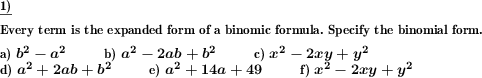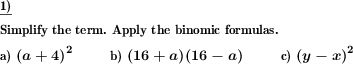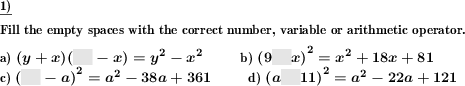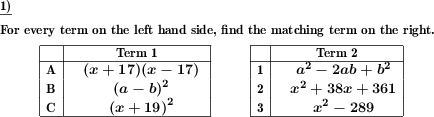Custom math worksheets at your fingertips# Details for problem "Specify binomic formula for expanded term"

Quickname: 6896

## Summary

A term is given which is the expanded form of a binomic formula. The original binomic formula has to be found.

## Example## Description

A term is presented. The term is the expanded form of one of the three binomic formulas. The task is to find the original binomic formula in the form of a product of two sums.

The summands of the formula's factors will be either a variable and a literal number or both of them will be variables. In no case, both summands will be numbers, which means the expanded term will always contain variables and it does not simplify to a single literal number.

The number of problems is selectable. For the types of formulas that will appear it can be specified that only some of the three binomic formulas will be used.

Variables that appear will always be named a and b or x and y.

Download free worksheets for this math problem here. The worksheet contains the problems only, the solutions sheet includes the answers. Just click on the respective link.

•Worksheet 1Solution sheet with answers
•Worksheet 2Solution sheet with answers
•Worksheet 3Solution sheet with answers

If you can not see the solution sheets for download, they may be filtered out by an ad blocker that you may have installed. If this is the case, please allow ads for this page and reload the page. The solution sheets will then reappear.

• Do the sample worksheets do not really fit?
• Do you need more math worksheets, with a different level of difficulty?
• Would you like to combine different problems on a worksheet and adjust them to your needs?
• As a teacher, you can put together your own worksheets using the automatically generated math problems provided.
With a free initial credit, you can start creating your own math worksheets in a few minutes.

It does not cost anything to try! Register here, to create custom worksheets now!

## Customization options for this problem

Parameter
Possible values
Number of problems
1, 2, 3, 4, 5, 6, 7, 8, 9, 10
Binomic formula types
(a+b)^2, (a-b)^2, (a+b)(a-b), (a+b)^2 & (a-b)^2, all

## Similar problems

Remark
Description
the other way round: Expand a binomic formula
Apply binomic formula expansion rules to simplify term.

## dw-Math worksheet templates that do contain this problem

Name
Title
Description
Binomic formulas: Expand and simplify
This worksheet provides basic exercises to become familiar with the binomic formulas.

## Other types of problems that appear on worksheets with this problem:

Relevance
Name
Description
Quickname
Example
****
Expand and simplify binomic formula
Apply binomic formula expansion rules to simplify term.****
Binomic formula fill in blanks
Fill gaps correctly in binomic formulas****
Match binomic formula to simplified form
Find matching terms of original and simplified binomic formulas in two lists.Deutsche Version dieser Aufgabe
These informational pages with samples describe math problems that can be combined on custom math worksheets with solutions for home and school use.
Deutsche Seiten
×